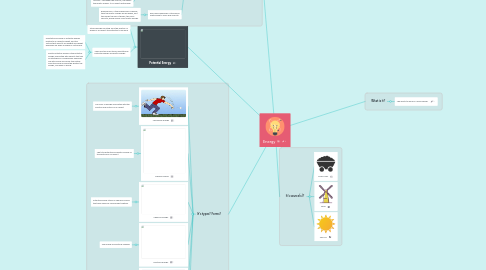# EnergySophia MAHIOUT
Get Started. It's FreeEnergy## 1. Potential Energy

### 1.2. There are two main types, gravitational potential energy and elastic energy.

1.2.1. Gravitational energy is potential energy related to an object's height, and the factors that affect it are weight and height. Examples are when a frisbee is motionless

1.2.2. Elastic potential energy is the potential energy associated with objects that can be stretched or compressed. Examples are with a bow and arrow; the farther back the arrow is pulled the greater the energy, and when a spring.

## 3. It's types? Forms?

### 3.1. Mechanical Energy

3.1.1. The form of energy associated with the position and motion of an object

### 3.2. Thermal Energy

3.2.1. The total potential and kinetic energy of the particles in an object

### 3.3. Chemical Energy

3.3.1. Potential energy stored in chemical bonds that hold chemical compounds together

### 3.4. Electrical Energy

3.4.1. The energy of electrical charges

### 3.5. Nuclear Energy

3.5.1. A type of potential energy stored in the nucleus of an atom.

### 3.6. Electromagnetic Energy

3.6.1. A type of energy that travels in waves and has some electrical and magnetic properties.

## 5. Classification?

### 5.1. Kinetic Energy

5.1.1. Energy in an object caused by motion.

5.1.2. You can calculate kinetic energy using the formula Kinetic energy = 1/2 x Mass x Velocity squared.

5.1.3. Factors Kinetic energy depends upon, and its relationship to each of those factors:

5.1.3.1. Mass- The higher the mass, the higher the kinetic energy, so there is a direct relationship.

5.1.3.2. Velocity- The higher the velocity, the higher the kinetic energy. It is a direct relationship.

5.1.4. Real World Examples of the above relationship to mass and velocity:

5.1.4.1. Bowling balls: If the bowling ball is heavier, then the kinetic energy will be higher, and the harder the ball is thrown, the more velocity, which means more kinetic energy.